Sandstorm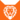狮族 (Q646)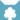天族 (Q632)[注 1]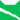雷族 武士天族 巫医[非正式][注 1]雷族 猫后雷族 武士雷族 长老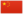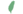沙崽非官 Sandkit  小沙非官
 沙爪 Sandpaw  沙掌
 沙风 Sandstorm  沙暴

Q717：雷族母猫，自《呼唤野性》登场的角色

## 细节

### 外貌

• 她是一只身形苗条、:179皮毛光滑:107柔软、:309:241叶绿色眼睛:158的淡姜色母猫，:猫物表身上有淡到几乎不可见的暗色条纹。:53

## 登场书目

• 呼唤野性 (Q57)[配角][首次登场🐱]
• 寒冰烈火 (Q59)[配角]
• 疑云重重 (Q60)[配角]
• 风起云涌 (Q65)[配角]
• 险路惊魂 (Q66)[配角]
• 力挽狂澜 (Q67)[配角]
• 午夜追踪 (Q139)[配角]
• 新月危机 (Q140)[配角]
• 重现家园 (Q141)[配角]
• 星光指路 (Q142)[配角]
• 黄昏战争 (Q143)[配角]
• 日落和平 (Q144)[配角]
• 预视力量 (Q152)[配角]
• 暗河汹涌 (Q153)[配角]
• 驱逐之战 (Q154)[配角]
• 天蚀遮月 (Q155)[配角]
• 暗夜长影 (Q156)[配角]
• 拂晓之光 (Q157)[配角]
• 第四学徒 (Q158)[配角]
• 战声渐近 (Q159)[配角]
• 暗夜密语 (Q160)[配角]
• 月光印记 (Q161)[配角]
• 武士归来 (Q162)[配角]
• 群星之战 (Q163)[配角]
• 学徒探索 (Q170)[配角][死亡时刻✝]
• 雷影交加 (Q171)[对话提及]
• 天空破碎 (Q172)[对话提及]
• 暗由心生 (Q4183)[对话提及]
• 火星的探索 (Q176)[主要角色]
• 蓝星的预言 (Q177)[配角]
• 天族的命运 (Q178)[对话提及]
• 黑莓星的风暴 (Q182)[配角]
• 鹰翅的旅程 (Q184)[对话提及]
• 鸦羽的拷问 (Q186)[猫物闲角]
• 松鼠飞的希冀 (Q3561)[配角]
• 灰条的誓言 (Q4057)[对话提及]
• 一星的告解 (Q4826)[配角]
• 冬青叶的故事 (Q187)[猫物闲角]
• 雾星的征兆 (Q188)[猫物闲角]
• 虎掌的愤怒 (Q190)[配角]
• 叶池的祈愿 (Q191)[配角]
• 鸽翅的沉默 (Q192)[配角]
• 乌爪的告别 (Q195)[对话提及]
• 红尾的恩债 (Q3565)[配角]
• 卵石光的幼崽 (Q3626)[对话提及]
• 蛾翅的秘密 (Q3628)[猫物闲角]
• 黑脚的审判 (Q4336)[猫物闲角]
• 变革之风 (Q4411)[配角]
• 武士失踪 (Q207)[仅于幻象]
• 和平破碎 (Q213)[仅于回忆]
• 族群救星 (Q214)[配角]
• 武士之心 (Q215)[配角]
• 紧急救援 (Q216)[对话提及]
• 洪水过后 (Q218)[对话提及]
• 族群的秘密 (Q199)[追溯修订]
• 族群的猫 (Q200)[配角]
• 族群的守则 (Q201)[配角]
• 族群的战争 (Q202)[配角]
• 终极指南 (Q204)[配角]
• 斑叶诚实的回答 (Q4319)[对话提及]
• 族群决定 (Q4580)[配角]
• 长老的忧虑 (Q4324)[对话提及]

更多资料：沙风/周边

## 脚注

1. 原因：天族重建 (Q3676) - 在《火星的探索》中，火星和沙风重建现代天族的过程
2. 尽管她并不是一名正式的巫医，但她在天族的时期中仍然根据她从炭毛学到的技能，起到了此作用。

## 参考文献

这篇文章基于CC BY-SA 3.0许可使用了猫武士维基（英语）Sandstorm一文中的部分内容。
1. 《学徒探索》第十四章 (Q1567)
2. 《呼唤野性》第一章 (Q741)
3. 某时间点 — 《呼唤野性》第一章 (Q741)
4. Official Website (2023-11-17). Sandstorm . WarriorCats.com .
5. Vicky Holmes (2009-08-25). 站内截图. 访问于 2022-05-26. “Graypaw's parents were Willowpelt and Patchpelt; Dustpaw and Ravenpaw's parents (yup, they were littermates! But Dustpaw NEVER admitted it!) were Robinwing and Fuzzypelt. And Sandpaw's folks were Brindleface and Redtail.”
6. 火星的探索 (Q176)午夜追踪 (Q139)
7. 风起云涌
8. 火星的探索
9. 力挽狂澜
10. 寒冰烈火
11. 呼唤野性
12. Su Susann (2016-09-30). 站内截图. Facebook . 原页面 归档于 2017-10-31. “As I said, Adderfang is a descandant of Spottedpelt and Pinestar is, trough the blood of his father, related to Gorseclaw.”
13. 星光指路
14. 紧急救援
15. 灰条的誓言